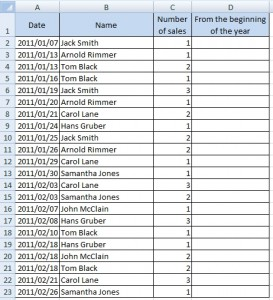# Running total for multiple items solved by array formula

There are some stuff dealers. In the following table is list of their sales. We need to know, how many sales they made from the beginning of the year. But there is a problem, we need to know sum to the date in each record for the dealer. For example: Jack Smith sold 1 piece on 2011/01/07 and then 3 pieces on 2011/01/19. So total to 2011/01/07 is 1, but total to 2011/01/19 is 4 (1+3).Question is how to create running total for each dealer in the table.

## Solution

This is array formula for the first record.

`{=SUM(IF(B2=\$B\$2:B2,\$C\$2:C2))}`

Note: Correct location of \$ character is important.1.How can I create a running total graph from this table?

2.Hi, This formula is simply amazing.. I had just an hiccup in adding one more condition to the IF.. say if you eg if the dealers had ‘on-field sales’ or ‘regular sales’ and I wanted to add that as an additional condition before cumulative computing, how do you do it?

Thanks for your revert in anticipation !!

3.I’ve tried this example, but there is a problem with the formula. It doesn’t calculate. Excel only writes {=SUM(IF(B2=\$B\$2:B2,\$C\$2:C2))}. What do I wrong?

•Hi, this is an array formula. Write just =SUM(IF(B2=\$B\$2:B2,\$C\$2:C2)) and press CTRL+SHIFT+ENTER.